In this lesson, we will continue to use the birth data in order to learn some basic plotting skills. Let’s start by reading the birth data into our Global Environment:

``births<-read.csv("births.csv",as.is=TRUE)``

## Line Plot

Let’s start by learning how to do a line plot. An informative line plot for this data would be to plot the average APGAR score for each by gestation period. Our intution would tell us that premature babies would have a lower APGAR score. Let’s see if this is correct. To check this, we first need to calculate the mean APGAR score for each of the levels of gestation period. We use the aggregate command to do this (aggregate is similar to a pivot table in Excel):

``apgar<-aggregate(APGAR5~ESTGEST,data=births,mean)``

Line charts are created with the function plot(x, y, type=) where x and y are numeric vectors of (x,y) points to connect. type= can take the following values:

type description
p points
l lines
o overplotted points and lines
b, c points (empty if “c”) joined by lines
s, S stair steps
h histogram-like vertical lines
n does not produce any points or lines

The points( ) function adds information to a graph. It can not produce a graph on its own. Usually it follows a plot(x, y) command that produces a graph.

Let’s plot the APGAR data:

``plot(apgar\$ESTGEST,apgar\$APGAR5)``This is generally what we expect, except we see there may be some errors with the data. Note that we have one data point with a gestation of 100 weeks. This would mean that the woman carried the baby for close to 25 months, which we assume is impossible. Let’s delete this data point and replot it. We also seem to have some bad data at the lower end of the spectrum. This is saying that we have a very healthy baby born at 12 weeks of gestation. This is also impossible.

``````apgar.sub<-subset(apgar,ESTGEST<60 & ESTGEST>17)
plot(apgar.sub\$ESTGEST,apgar.sub\$APGAR5)``````Now let’s dress up our plot:

``plot(apgar.sub\$ESTGEST,apgar.sub\$APGAR5,type="l",col="blue",xlab="Estimated Gestation",ylab="APGARS",main="Mean APGARS vs. Gestation")``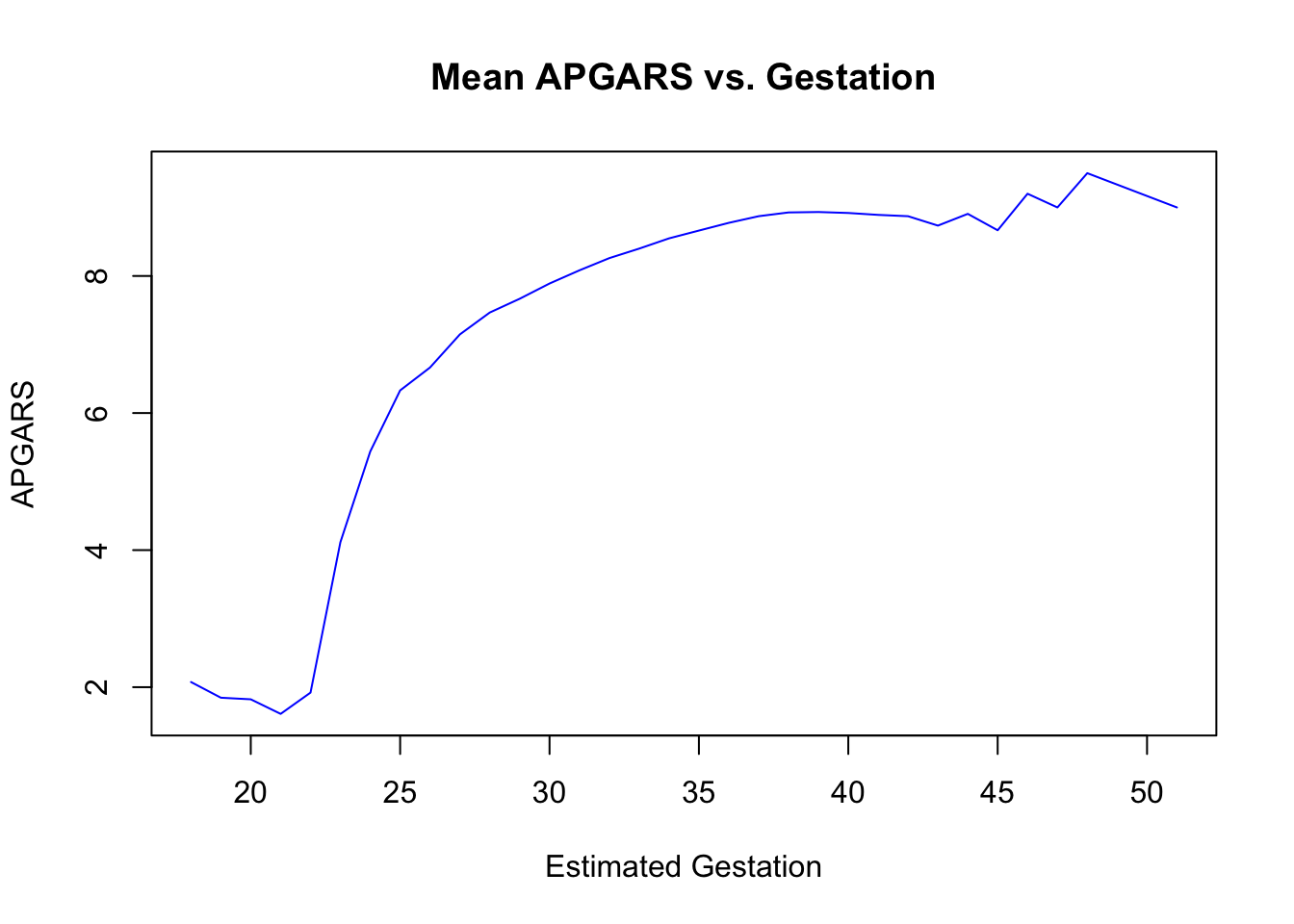Now let’s explore weight gain as a function of age:

``````wt<-aggregate(WTGAIN~MAGER,data=births,mean)
plot(wt\$MAGER,wt\$WTGAIN,type="l",col="red",xlab="Age",ylab="Weight Gain",main="Weight Gain vs. Age")``````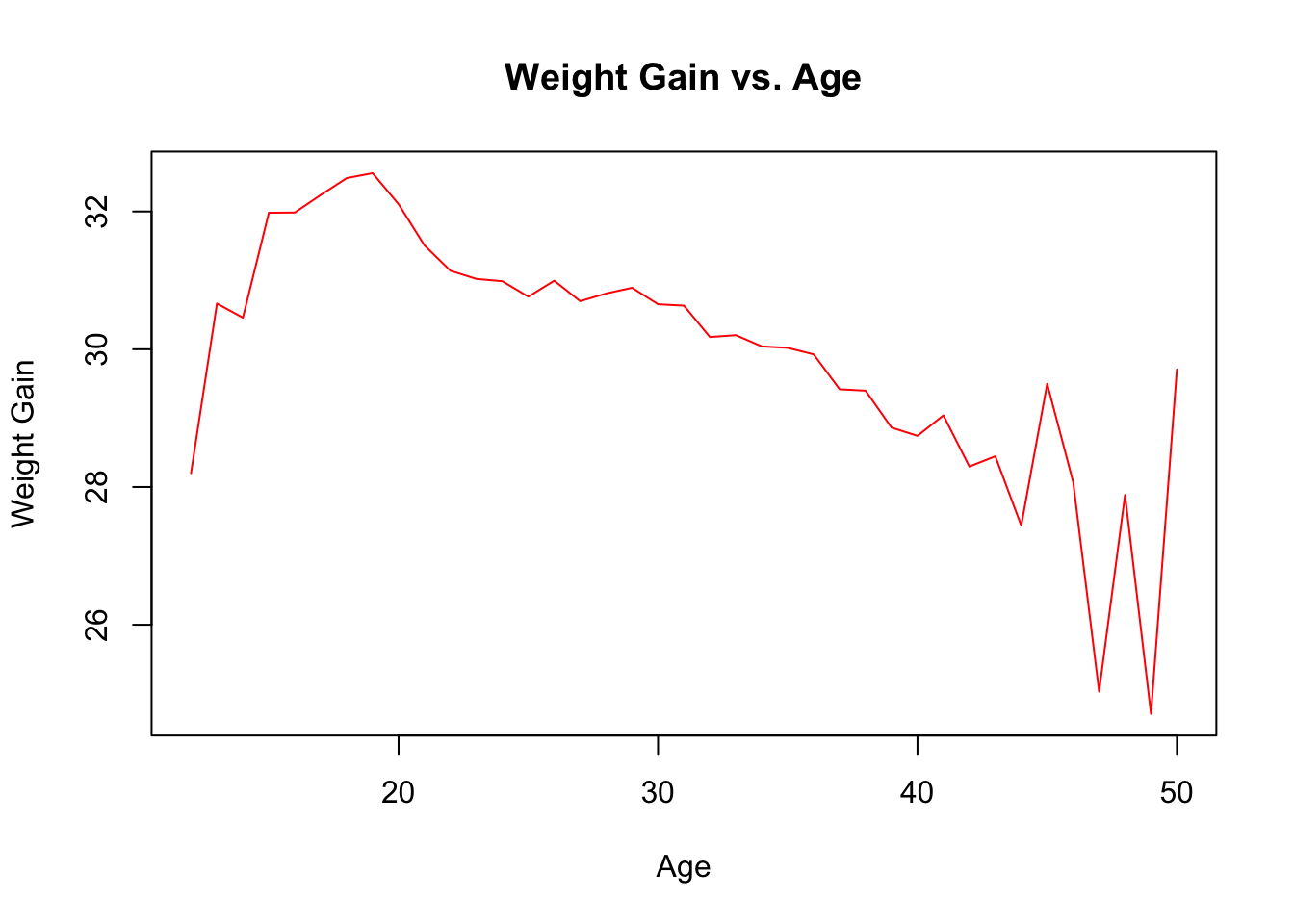## Bar Plot

Next we’ll use a barplot to explore month of birth. Remember that we use the table command inside of the barplot command:

``barplot(table(births\$DOB_MM))``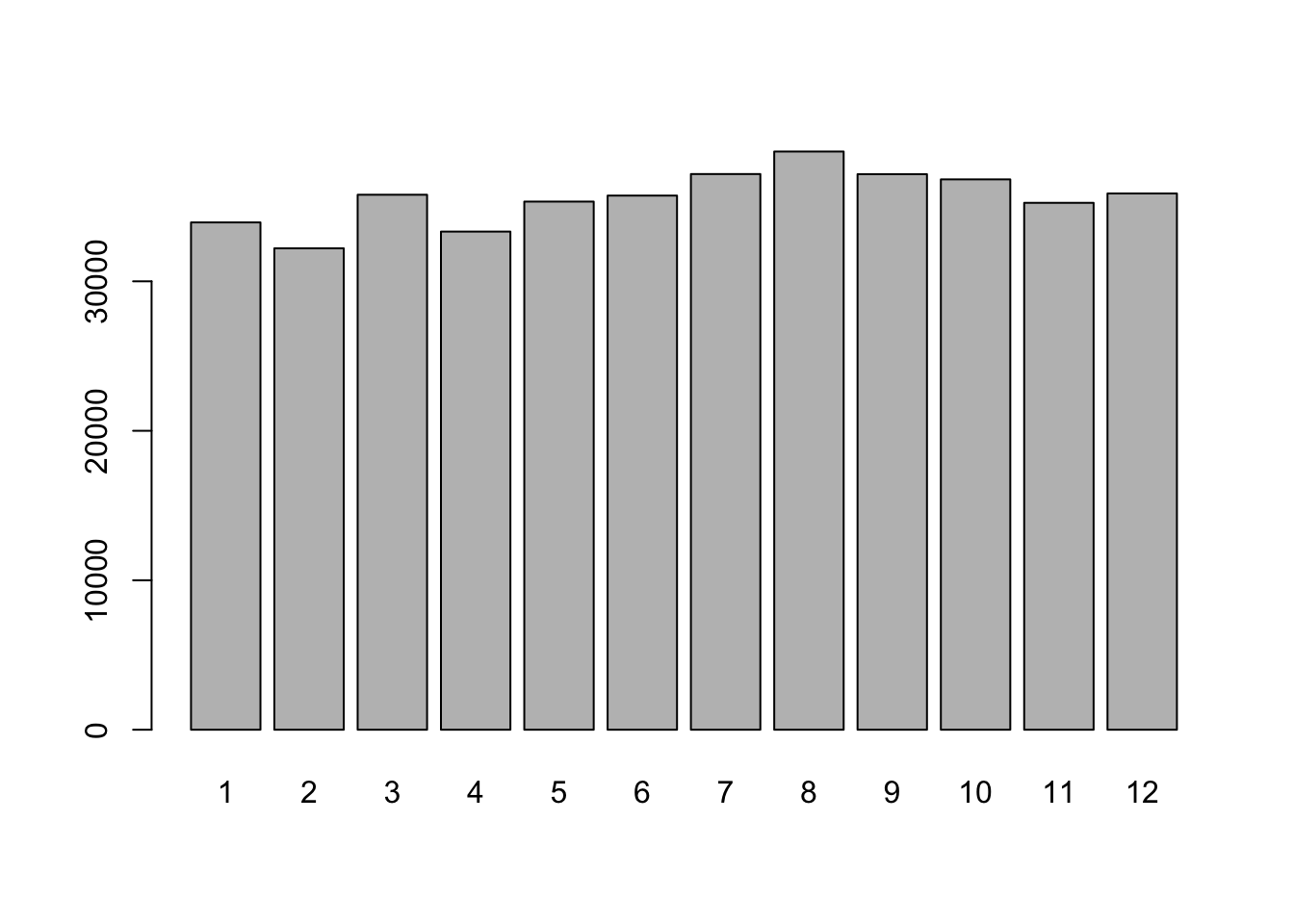Looks like we have a peak in late summer. Let’s dress ths plot up a bit:

``barplot(table(births\$DOB_MM),col=rainbow(12),main="Number of Births by Month")``Note that the rainbow(12) command grabs 12 colors from a rainbow palette. A similar palette is the heat.colors palette:

``barplot(table(births\$DOB_MM),col=heat.colors(12),main="Number of Births by Month")``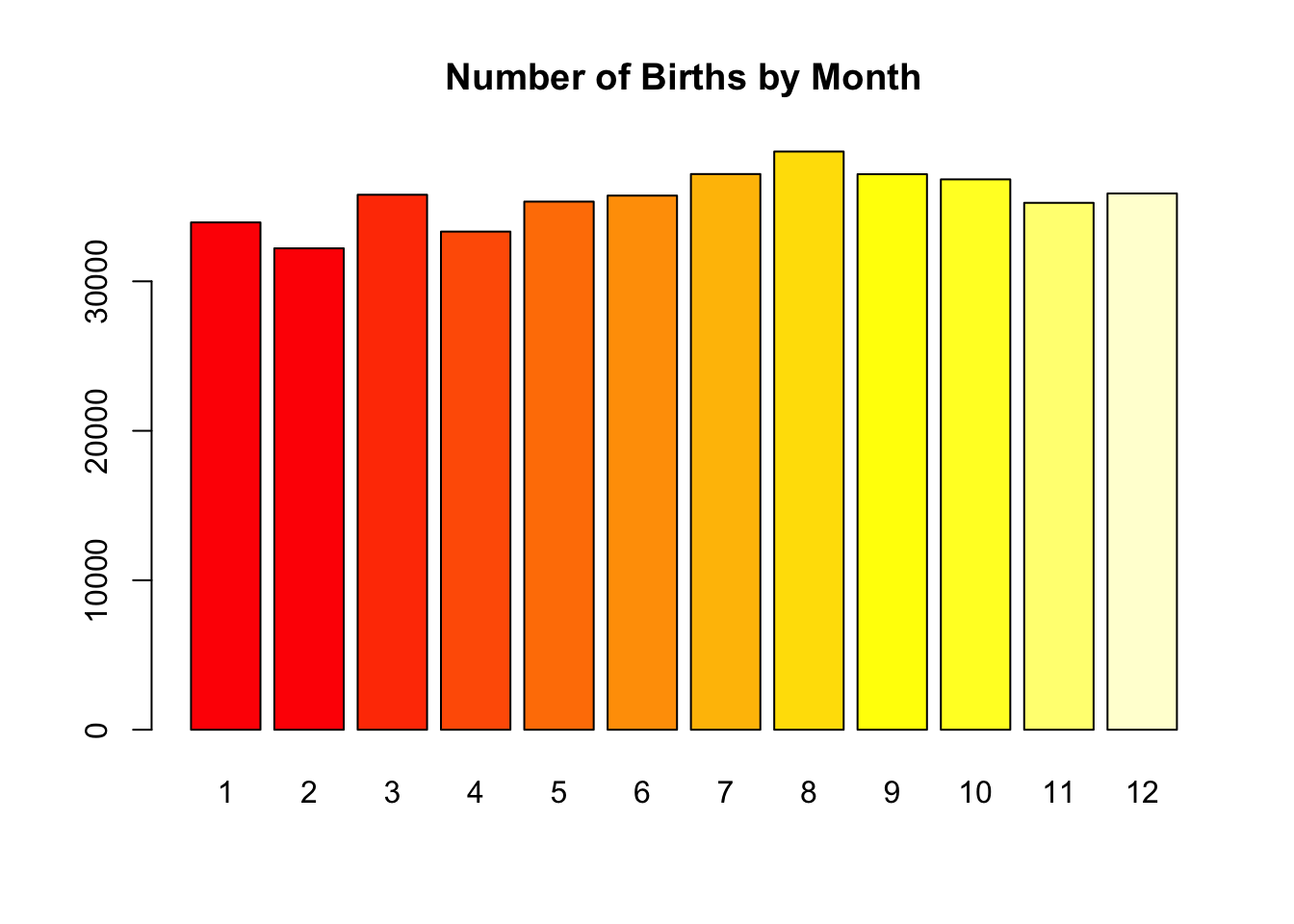Now let’s see if births happen a certain day of the week:

``barplot(table(births\$DOB_WK),col=rainbow(12),main="Number of Births by Month")``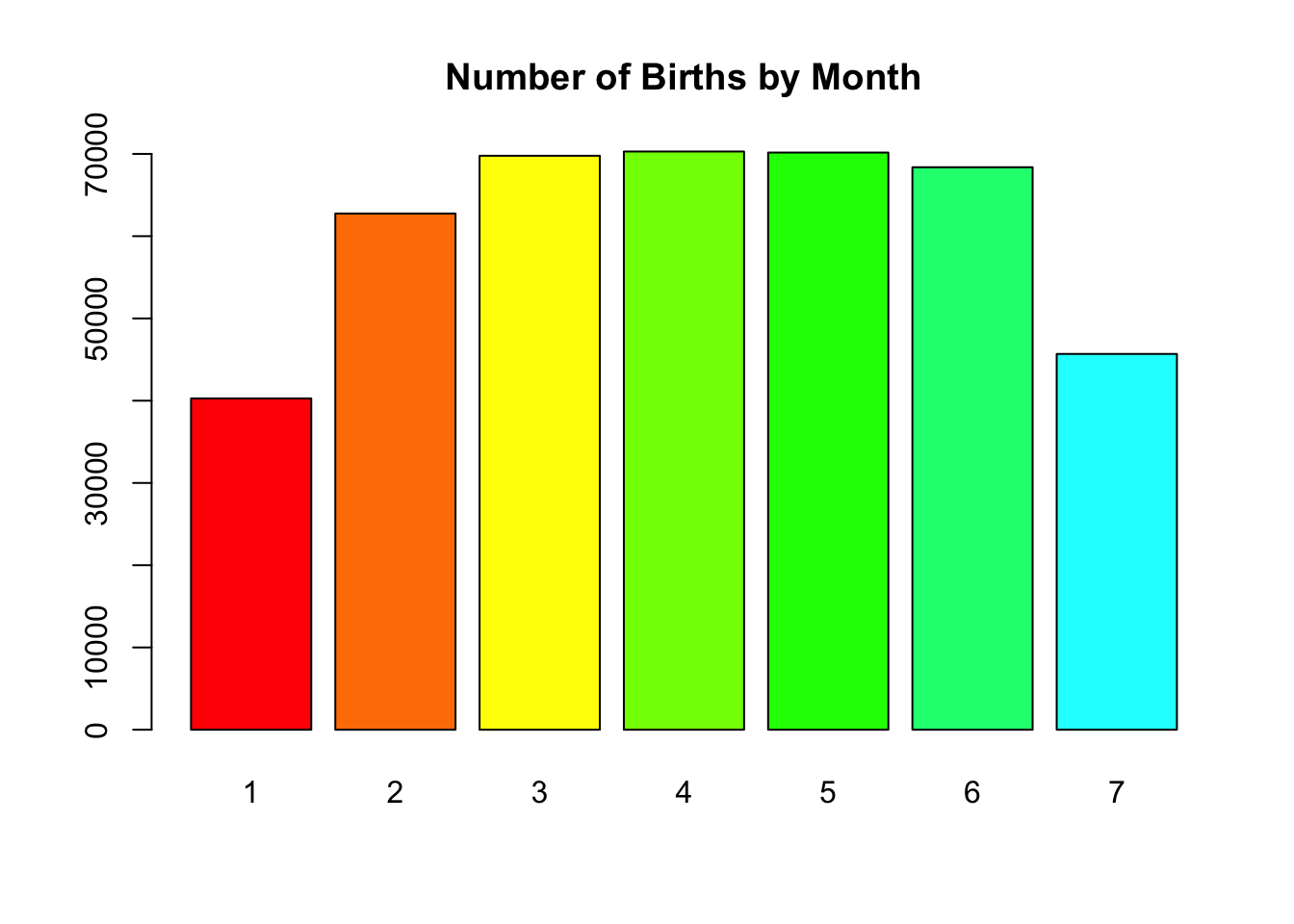It looks like babies are generally not born on weekends.

## Pie Plot

Here’s how to make a pie plot of the gender of the newborn babies:

``pie(table(births\$SEX),main="Gender of Newborn",col=c("red","blue"))``